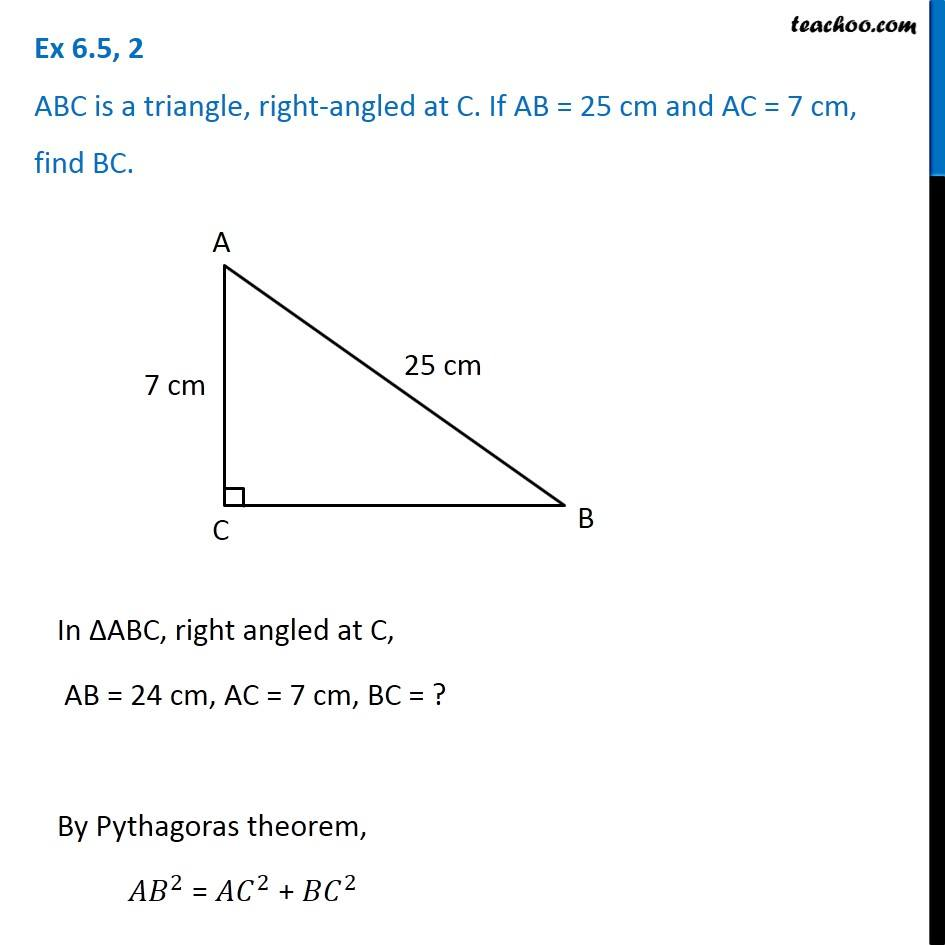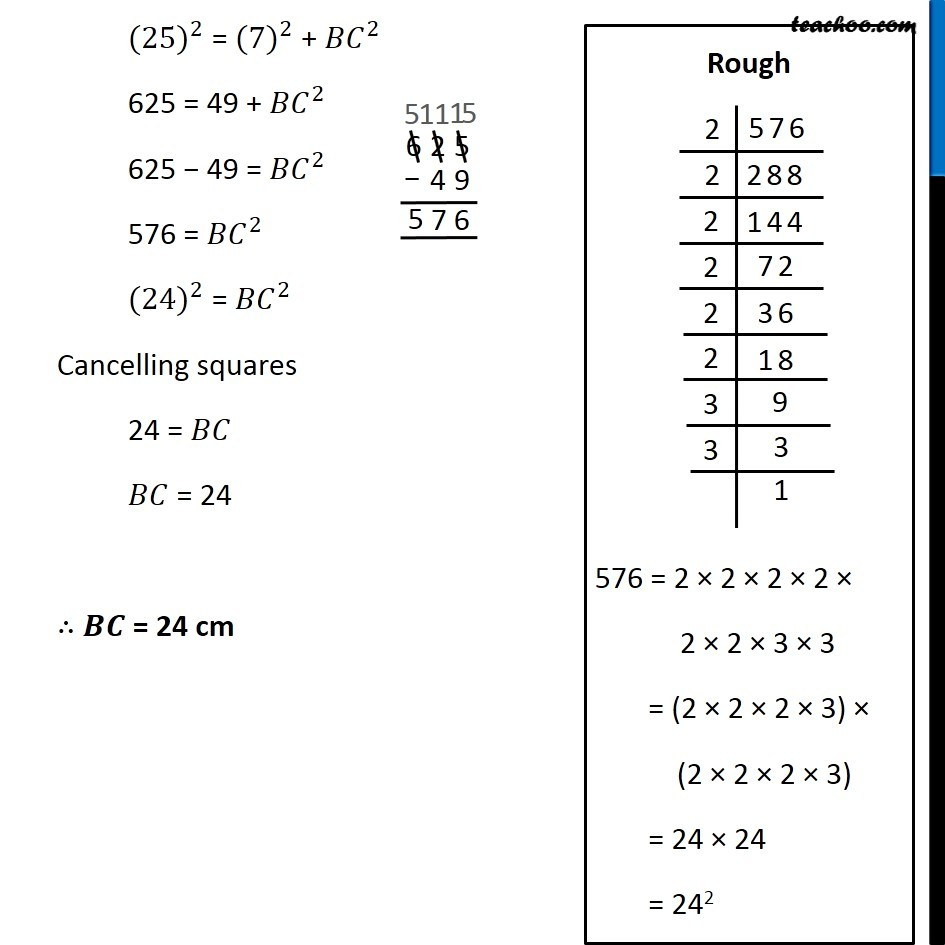1. Chapter 6 Class 7 Triangle and its Properties
2. Concept wise
3. Pythagoras Theorem

Transcript

Ex 6.5, 2 ABC is a triangle, right-angled at C. If AB = 25 cm and AC = 7 cm, find BC.In ∆ABC, right angled at C, AB = 24 cm, AC = 7 cm, BC = ? By Pythagoras theorem, 〖𝐴𝐵〗^2 = 〖𝐴𝐶〗^2 + 〖𝐵𝐶〗^2 〖(25)〗^2 = 〖(7)〗^2 + 〖𝐵𝐶〗^2 625 = 49 + 〖𝐵𝐶〗^2 625 − 49 = 〖𝐵𝐶〗^2 576 = 〖𝐵𝐶〗^2 〖(24)〗^2 = 〖𝐵𝐶〗^2 Cancelling squares 24 = 𝐵𝐶 𝐵𝐶 = 24 ∴ 𝑩𝑪 = 24 cm Rough 576 = 2 × 2 × 2 × 2 × 2 × 2 × 3 × 3 = (2 × 2 × 2 × 3) × (2 × 2 × 2 × 3) = 24 × 24 = 242

Pythagoras Theorem